# QPainterPath Class

The QPainterPath class provides a container for painting operations, enabling graphical shapes to be constructed and reused. More...

 Header: #include qmake: QT += gui

## Public Types

 class Element enum ElementType { MoveToElement, LineToElement, CurveToElement, CurveToDataElement }

## Public Functions

 QDataStream & operator<<(QDataStream &stream, const QPainterPath &path) QDataStream & operator>>(QDataStream &stream, QPainterPath &path)

## Detailed Description

A painter path is an object composed of a number of graphical building blocks, such as rectangles, ellipses, lines, and curves. Building blocks can be joined in closed subpaths, for example as a rectangle or an ellipse. A closed path has coinciding start and end points. Or they can exist independently as unclosed subpaths, such as lines and curves.

A QPainterPath object can be used for filling, outlining, and clipping. To generate fillable outlines for a given painter path, use the QPainterPathStroker class. The main advantage of painter paths over normal drawing operations is that complex shapes only need to be created once; then they can be drawn many times using only calls to the QPainter::drawPath() function.

QPainterPath provides a collection of functions that can be used to obtain information about the path and its elements. In addition it is possible to reverse the order of the elements using the toReversed() function. There are also several functions to convert this painter path object into a polygon representation.

### Composing a QPainterPath

A QPainterPath object can be constructed as an empty path, with a given start point, or as a copy of another QPainterPath object. Once created, lines and curves can be added to the path using the lineTo(), arcTo(), cubicTo() and quadTo() functions. The lines and curves stretch from the currentPosition() to the position passed as argument.

The currentPosition() of the QPainterPath object is always the end position of the last subpath that was added (or the initial start point). Use the moveTo() function to move the currentPosition() without adding a component. The moveTo() function implicitly starts a new subpath, and closes the previous one. Another way of starting a new subpath is to call the closeSubpath() function which closes the current path by adding a line from the currentPosition() back to the path's start position. Note that the new path will have (0, 0) as its initial currentPosition().

In addition, a path can be added to the current path using the connectPath() function. But note that this function will connect the last element of the current path to the first element of given one by adding a line.

Below is a code snippet that shows how a QPainterPath object can be used: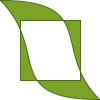```QPainterPath path; path.addRect(20, 20, 60, 60); path.moveTo(0, 0); path.cubicTo(99, 0, 50, 50, 99, 99); path.cubicTo(0, 99, 50, 50, 0, 0); QPainter painter(this); painter.fillRect(0, 0, 100, 100, Qt::white); painter.setPen(QPen(QColor(79, 106, 25), 1, Qt::SolidLine, Qt::FlatCap, Qt::MiterJoin)); painter.setBrush(QColor(122, 163, 39)); painter.drawPath(path);```

The painter path is initially empty when constructed. We first add a rectangle, which is a closed subpath. Then we add two bezier curves which together form a closed subpath even though they are not closed individually. Finally we draw the entire path. The path is filled using the default fill rule, Qt::OddEvenFill. Qt provides two methods for filling paths:

Qt::OddEvenFillQt::WindingFill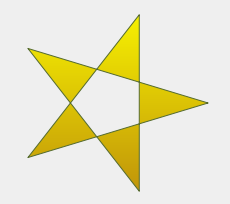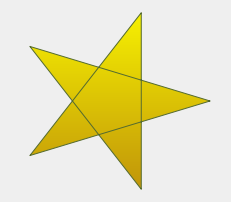See the Qt::FillRule documentation for the definition of the rules. A painter path's currently set fill rule can be retrieved using the fillRule() function, and altered using the setFillRule() function.

### QPainterPath Information

The QPainterPath class provides a collection of functions that returns information about the path and its elements.

The currentPosition() function returns the end point of the last subpath that was added (or the initial start point). The elementAt() function can be used to retrieve the various subpath elements, the number of elements can be retrieved using the elementCount() function, and the isEmpty() function tells whether this QPainterPath object contains any elements at all.

The controlPointRect() function returns the rectangle containing all the points and control points in this path. This function is significantly faster to compute than the exact boundingRect() which returns the bounding rectangle of this painter path with floating point precision.

Finally, QPainterPath provides the contains() function which can be used to determine whether a given point or rectangle is inside the path, and the intersects() function which determines if any of the points inside a given rectangle also are inside this path.

### QPainterPath Conversion

For compatibility reasons, it might be required to simplify the representation of a painter path: QPainterPath provides the toFillPolygon(), toFillPolygons() and toSubpathPolygons() functions which convert the painter path into a polygon. The toFillPolygon() returns the painter path as one single polygon, while the two latter functions return a list of polygons.

The toFillPolygons() and toSubpathPolygons() functions are provided because it is usually faster to draw several small polygons than to draw one large polygon, even though the total number of points drawn is the same. The difference between the two is the number of polygons they return: The toSubpathPolygons() creates one polygon for each subpath regardless of intersecting subpaths (i.e. overlapping bounding rectangles), while the toFillPolygons() functions creates only one polygon for overlapping subpaths.

The toFillPolygon() and toFillPolygons() functions first convert all the subpaths to polygons, then uses a rewinding technique to make sure that overlapping subpaths can be filled using the correct fill rule. Note that rewinding inserts additional lines in the polygon so the outline of the fill polygon does not match the outline of the path.

### Examples

Qt provides the Painter Paths Example and the Vector Deformation example which are located in Qt's example directory.

The Painter Paths Example shows how painter paths can be used to build complex shapes for rendering and lets the user experiment with the filling and stroking. The Vector Deformation Example shows how to use QPainterPath to draw text.

Painter Paths ExampleVector Deformation Example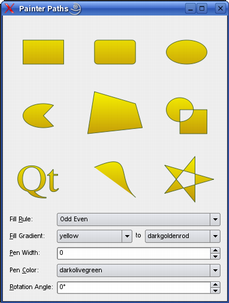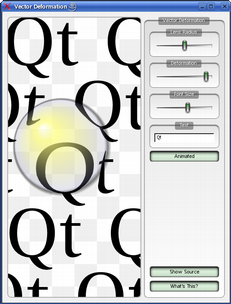## Member Type Documentation

### enum QPainterPath::ElementType

This enum describes the types of elements used to connect vertices in subpaths.

ConstantValueDescription
`QPainterPath::MoveToElement``0`A new subpath. See also moveTo().
`QPainterPath::LineToElement``1`A line. See also lineTo().
`QPainterPath::CurveToElement``2`A curve. See also cubicTo() and quadTo().
`QPainterPath::CurveToDataElement``3`The extra data required to describe a curve in a CurveToElement element.

## Member Function Documentation

### QPainterPath::QPainterPath(const QPainterPath &path)

Creates a QPainterPath object that is a copy of the given path.

### QPainterPath::QPainterPath(const QPointF &startPoint)

Creates a QPainterPath object with the given startPoint as its current position.

### QPainterPath::QPainterPath()

Constructs an empty QPainterPath object.

### QPainterPath &QPainterPath::operator=(QPainterPath &&other)

Move-assigns other to this QPainterPath instance.

This function was introduced in Qt 5.2.

### QPainterPath &QPainterPath::operator=(const QPainterPath &path)

Assigns the given path to this painter path.

### QPainterPath::~QPainterPath()

Destroys this QPainterPath object.

Creates an ellipse within the specified boundingRectangle and adds it to the painter path as a closed subpath.

The ellipse is composed of a clockwise curve, starting and finishing at zero degrees (the 3 o'clock position).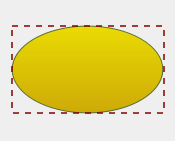```QLinearGradient myGradient; QPen myPen; QRectF boundingRectangle; QPainterPath myPath; myPath.addEllipse(boundingRectangle); QPainter painter(this); painter.setBrush(myGradient); painter.setPen(myPen); painter.drawPath(myPath);```

### void QPainterPath::addEllipse(qrealx, qrealy, qrealwidth, qrealheight)

Creates an ellipse within the bounding rectangle defined by its top-left corner at (x, y), width and height, and adds it to the painter path as a closed subpath.

### void QPainterPath::addEllipse(const QPointF &center, qrealrx, qrealry)

Creates an ellipse positioned at center with radii rx and ry, and adds it to the painter path as a closed subpath.

This function was introduced in Qt 4.4.

Adds the given path to this path as a closed subpath.

Adds the given polygon to the path as an (unclosed) subpath.

Note that the current position after the polygon has been added, is the last point in polygon. To draw a line back to the first point, use the closeSubpath() function.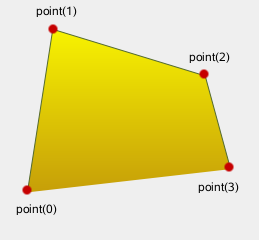```QLinearGradient myGradient; QPen myPen; QPolygonF myPolygon; QPainterPath myPath; myPath.addPolygon(myPolygon); QPainter painter(this); painter.setBrush(myGradient); painter.setPen(myPen); painter.drawPath(myPath);```

Adds the given rectangle to this path as a closed subpath.

The rectangle is added as a clockwise set of lines. The painter path's current position after the rectangle has been added is at the top-left corner of the rectangle.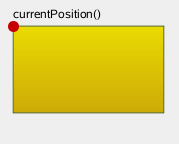```QLinearGradient myGradient; QPen myPen; QRectF myRectangle; QPainterPath myPath; myPath.addRect(myRectangle); QPainter painter(this); painter.setBrush(myGradient); painter.setPen(myPen); painter.drawPath(myPath);```

### void QPainterPath::addRect(qrealx, qrealy, qrealwidth, qrealheight)

Adds a rectangle at position (x, y), with the given width and height, as a closed subpath.

Adds the given region to the path by adding each rectangle in the region as a separate closed subpath.

Adds the given rectangle rect with rounded corners to the path.

The xRadius and yRadius arguments specify the radii of the ellipses defining the corners of the rounded rectangle. When mode is Qt::RelativeSize, xRadius and yRadius are specified in percentage of half the rectangle's width and height respectively, and should be in the range 0.0 to 100.0.

This function was introduced in Qt 4.4.

Adds the given rectangle x, y, w, h with rounded corners to the path.

This function was introduced in Qt 4.4.

### void QPainterPath::addText(const QPointF &point, const QFont &font, const QString &text)

Adds the given text to this path as a set of closed subpaths created from the font supplied. The subpaths are positioned so that the left end of the text's baseline lies at the specified point.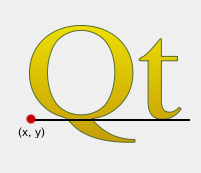```QLinearGradient myGradient; QPen myPen; QFont myFont; QPointF baseline(x, y); QPainterPath myPath; myPath.addText(baseline, myFont, tr("Qt")); QPainter painter(this); painter.setBrush(myGradient); painter.setPen(myPen); painter.drawPath(myPath);```

### void QPainterPath::addText(qrealx, qrealy, const QFont &font, const QString &text)

Adds the given text to this path as a set of closed subpaths created from the font supplied. The subpaths are positioned so that the left end of the text's baseline lies at the point specified by (x, y).

### qreal QPainterPath::angleAtPercent(qrealt) const

Returns the angle of the path tangent at the percentage t. The argument t has to be between 0 and 1.

Positive values for the angles mean counter-clockwise while negative values mean the clockwise direction. Zero degrees is at the 3 o'clock position.

Note that similarly to the other percent methods, the percentage measurement is not linear with regards to the length if curves are present in the path. When curves are present the percentage argument is mapped to the t parameter of the Bezier equations.

### void QPainterPath::arcMoveTo(const QRectF &rectangle, qrealangle)

Creates a move to that lies on the arc that occupies the given rectangle at angle.

Angles are specified in degrees. Clockwise arcs can be specified using negative angles.

This function was introduced in Qt 4.2.

### void QPainterPath::arcMoveTo(qrealx, qrealy, qrealwidth, qrealheight, qrealangle)

Creates a move to that lies on the arc that occupies the QRectF(x, y, width, height) at angle.

This function was introduced in Qt 4.2.

### void QPainterPath::arcTo(const QRectF &rectangle, qrealstartAngle, qrealsweepLength)

Creates an arc that occupies the given rectangle, beginning at the specified startAngle and extending sweepLength degrees counter-clockwise.

Angles are specified in degrees. Clockwise arcs can be specified using negative angles.

Note that this function connects the starting point of the arc to the current position if they are not already connected. After the arc has been added, the current position is the last point in arc. To draw a line back to the first point, use the closeSubpath() function.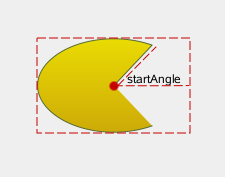```QLinearGradient myGradient; QPen myPen; QPointF center, startPoint; QPainterPath myPath; myPath.moveTo(center); myPath.arcTo(boundingRect, startAngle, sweepLength); QPainter painter(this); painter.setBrush(myGradient); painter.setPen(myPen); painter.drawPath(myPath);```

### void QPainterPath::arcTo(qrealx, qrealy, qrealwidth, qrealheight, qrealstartAngle, qrealsweepLength)

Creates an arc that occupies the rectangle QRectF(x, y, width, height), beginning at the specified startAngle and extending sweepLength degrees counter-clockwise.

### QRectF QPainterPath::boundingRect() const

Returns the bounding rectangle of this painter path as a rectangle with floating point precision.

### int QPainterPath::capacity() const

Returns the number of elements allocated by the QPainterPath.

This function was introduced in Qt 5.13.

Clears the path elements stored.

This allows the path to reuse previous memory allocations.

This function was introduced in Qt 5.13.

### void QPainterPath::closeSubpath()

Closes the current subpath by drawing a line to the beginning of the subpath, automatically starting a new path. The current point of the new path is (0, 0).

If the subpath does not contain any elements, this function does nothing.

### void QPainterPath::connectPath(const QPainterPath &path)

Connects the given path to this path by adding a line from the last element of this path to the first element of the given path.

### bool QPainterPath::contains(const QPointF &point) const

Returns `true` if the given point is inside the path, otherwise returns `false`.

### bool QPainterPath::contains(const QRectF &rectangle) const

Returns `true` if the given rectangle is inside the path, otherwise returns `false`.

### bool QPainterPath::contains(const QPainterPath &p) const

Returns `true` if the given path p is contained within the current path. Returns `false` if any edges of the current path and p intersect.

Set operations on paths will treat the paths as areas. Non-closed paths will be treated as implicitly closed.

This function was introduced in Qt 4.3.

### QRectF QPainterPath::controlPointRect() const

Returns the rectangle containing all the points and control points in this path.

This function is significantly faster to compute than the exact boundingRect(), and the returned rectangle is always a superset of the rectangle returned by boundingRect().

### void QPainterPath::cubicTo(const QPointF &c1, const QPointF &c2, const QPointF &endPoint)

Adds a cubic Bezier curve between the current position and the given endPoint using the control points specified by c1, and c2.

After the curve is added, the current position is updated to be at the end point of the curve.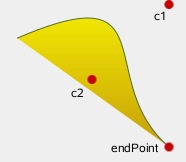```QLinearGradient myGradient; QPen myPen; QPainterPath myPath; myPath.cubicTo(c1, c2, endPoint); QPainter painter(this); painter.setBrush(myGradient); painter.setPen(myPen); painter.drawPath(myPath);```

### void QPainterPath::cubicTo(qrealc1X, qrealc1Y, qrealc2X, qrealc2Y, qrealendPointX, qrealendPointY)

Adds a cubic Bezier curve between the current position and the end point (endPointX, endPointY) with control points specified by (c1X, c1Y) and (c2X, c2Y).

### QPointF QPainterPath::currentPosition() const

Returns the current position of the path.

### QPainterPath::Element QPainterPath::elementAt(intindex) const

Returns the element at the given index in the painter path.

### int QPainterPath::elementCount() const

Returns the number of path elements in the painter path.

### Qt::FillRule QPainterPath::fillRule() const

Returns the painter path's currently set fill rule.

### QPainterPath QPainterPath::intersected(const QPainterPath &p) const

Returns a path which is the intersection of this path's fill area and p's fill area. Bezier curves may be flattened to line segments due to numerical instability of doing bezier curve intersections.

This function was introduced in Qt 4.3.

### bool QPainterPath::intersects(const QRectF &rectangle) const

Returns `true` if any point in the given rectangle intersects the path; otherwise returns `false`.

There is an intersection if any of the lines making up the rectangle crosses a part of the path or if any part of the rectangle overlaps with any area enclosed by the path. This function respects the current fillRule to determine what is considered inside the path.

### bool QPainterPath::intersects(const QPainterPath &p) const

Returns `true` if the current path intersects at any point the given path p. Also returns `true` if the current path contains or is contained by any part of p.

Set operations on paths will treat the paths as areas. Non-closed paths will be treated as implicitly closed.

This function was introduced in Qt 4.3.

### bool QPainterPath::isEmpty() const

Returns `true` if either there are no elements in this path, or if the only element is a MoveToElement; otherwise returns `false`.

### qreal QPainterPath::length() const

Returns the length of the current path.

### void QPainterPath::lineTo(const QPointF &endPoint)

Adds a straight line from the current position to the given endPoint. After the line is drawn, the current position is updated to be at the end point of the line.

### void QPainterPath::lineTo(qrealx, qrealy)

Draws a line from the current position to the point (x, y).

### void QPainterPath::moveTo(const QPointF &point)

Moves the current point to the given point, implicitly starting a new subpath and closing the previous one.

### void QPainterPath::moveTo(qrealx, qrealy)

Moves the current position to (x, y) and starts a new subpath, implicitly closing the previous path.

### qreal QPainterPath::percentAtLength(qreallen) const

Returns percentage of the whole path at the specified length len.

Note that similarly to other percent methods, the percentage measurement is not linear with regards to the length, if curves are present in the path. When curves are present the percentage argument is mapped to the t parameter of the Bezier equations.

### QPointF QPainterPath::pointAtPercent(qrealt) const

Returns the point at at the percentage t of the current path. The argument t has to be between 0 and 1.

Note that similarly to other percent methods, the percentage measurement is not linear with regards to the length, if curves are present in the path. When curves are present the percentage argument is mapped to the t parameter of the Bezier equations.

### void QPainterPath::quadTo(const QPointF &c, const QPointF &endPoint)

Adds a quadratic Bezier curve between the current position and the given endPoint with the control point specified by c.

After the curve is added, the current point is updated to be at the end point of the curve.

### void QPainterPath::quadTo(qrealcx, qrealcy, qrealendPointX, qrealendPointY)

Adds a quadratic Bezier curve between the current point and the endpoint (endPointX, endPointY) with the control point specified by (cx, cy).

### void QPainterPath::reserve(intsize)

Reserves a given amount of elements in QPainterPath's internal memory.

Attempts to allocate memory for at least size elements.

This function was introduced in Qt 5.13.

### void QPainterPath::setElementPositionAt(intindex, qrealx, qrealy)

Sets the x and y coordinate of the element at index index to x and y.

This function was introduced in Qt 4.2.

### void QPainterPath::setFillRule(Qt::FillRulefillRule)

Sets the fill rule of the painter path to the given fillRule. Qt provides two methods for filling paths:

Qt::OddEvenFill (default)Qt::WindingFill### QPainterPath QPainterPath::simplified() const

Returns a simplified version of this path. This implies merging all subpaths that intersect, and returning a path containing no intersecting edges. Consecutive parallel lines will also be merged. The simplified path will always use the default fill rule, Qt::OddEvenFill. Bezier curves may be flattened to line segments due to numerical instability of doing bezier curve intersections.

This function was introduced in Qt 4.4.

### qreal QPainterPath::slopeAtPercent(qrealt) const

Returns the slope of the path at the percentage t. The argument t has to be between 0 and 1.

Note that similarly to other percent methods, the percentage measurement is not linear with regards to the length, if curves are present in the path. When curves are present the percentage argument is mapped to the t parameter of the Bezier equations.

### QPainterPath QPainterPath::subtracted(const QPainterPath &p) const

Returns a path which is p's fill area subtracted from this path's fill area.

Set operations on paths will treat the paths as areas. Non-closed paths will be treated as implicitly closed. Bezier curves may be flattened to line segments due to numerical instability of doing bezier curve intersections.

This function was introduced in Qt 4.3.

### void QPainterPath::swap(QPainterPath &other)

Swaps painter path other with this painter path. This operation is very fast and never fails.

This function was introduced in Qt 4.8.

### QPolygonF QPainterPath::toFillPolygon(const QTransform &matrix = QTransform()) const

Converts the path into a polygon using the QTransform matrix, and returns the polygon.

The polygon is created by first converting all subpaths to polygons, then using a rewinding technique to make sure that overlapping subpaths can be filled using the correct fill rule.

Note that rewinding inserts addition lines in the polygon so the outline of the fill polygon does not match the outline of the path.

### QList<QPolygonF> QPainterPath::toFillPolygons(const QTransform &matrix = QTransform()) const

Converts the path into a list of polygons using the QTransform matrix, and returns the list.

The function differs from the toFillPolygon() function in that it creates several polygons. It is provided because it is usually faster to draw several small polygons than to draw one large polygon, even though the total number of points drawn is the same.

The toFillPolygons() function differs from the toSubpathPolygons() function in that it create only polygon for subpaths that have overlapping bounding rectangles.

Like the toFillPolygon() function, this function uses a rewinding technique to make sure that overlapping subpaths can be filled using the correct fill rule. Note that rewinding inserts addition lines in the polygons so the outline of the fill polygon does not match the outline of the path.

### QPainterPath QPainterPath::toReversed() const

Creates and returns a reversed copy of the path.

It is the order of the elements that is reversed: If a QPainterPath is composed by calling the moveTo(), lineTo() and cubicTo() functions in the specified order, the reversed copy is composed by calling cubicTo(), lineTo() and moveTo().

### QList<QPolygonF> QPainterPath::toSubpathPolygons(const QTransform &matrix = QTransform()) const

Converts the path into a list of polygons using the QTransform matrix, and returns the list.

This function creates one polygon for each subpath regardless of intersecting subpaths (i.e. overlapping bounding rectangles). To make sure that such overlapping subpaths are filled correctly, use the toFillPolygons() function instead.

### void QPainterPath::translate(qrealdx, qrealdy)

Translates all elements in the path by (dx, dy).

This function was introduced in Qt 4.6.

### void QPainterPath::translate(const QPointF &offset)

Translates all elements in the path by the given offset.

This function was introduced in Qt 4.6.

### QPainterPath QPainterPath::translated(qrealdx, qrealdy) const

Returns a copy of the path that is translated by (dx, dy).

This function was introduced in Qt 4.6.

### QPainterPath QPainterPath::translated(const QPointF &offset) const

Returns a copy of the path that is translated by the given offset.

This function was introduced in Qt 4.6.

### QPainterPath QPainterPath::united(const QPainterPath &p) const

Returns a path which is the union of this path's fill area and p's fill area.

Set operations on paths will treat the paths as areas. Non-closed paths will be treated as implicitly closed. Bezier curves may be flattened to line segments due to numerical instability of doing bezier curve intersections.

This function was introduced in Qt 4.3.

### bool QPainterPath::operator!=(const QPainterPath &path) const

Returns `true` if this painter path differs from the given path.

Note that comparing paths may involve a per element comparison which can be slow for complex paths.

### QPainterPath QPainterPath::operator&(const QPainterPath &other) const

Returns the intersection of this path and the other path.

This function was introduced in Qt 4.5.

### QPainterPath &QPainterPath::operator&=(const QPainterPath &other)

Intersects this path with other and returns a reference to this path.

This function was introduced in Qt 4.5.

### QPainterPath QPainterPath::operator+(const QPainterPath &other) const

Returns the union of this path and the other path. This function is equivalent to operator|().

This function was introduced in Qt 4.5.

### QPainterPath &QPainterPath::operator+=(const QPainterPath &other)

Unites this path with other, and returns a reference to this path. This is equivalent to operator|=().

This function was introduced in Qt 4.5.

### QPainterPath QPainterPath::operator-(const QPainterPath &other) const

Subtracts the other path from a copy of this path, and returns the copy.

This function was introduced in Qt 4.5.

### QPainterPath &QPainterPath::operator-=(const QPainterPath &other)

Subtracts other from this path, and returns a reference to this path.

This function was introduced in Qt 4.5.

### bool QPainterPath::operator==(const QPainterPath &path) const

Returns `true` if this painterpath is equal to the given path.

Note that comparing paths may involve a per element comparison which can be slow for complex paths.

### QPainterPath QPainterPath::operator|(const QPainterPath &other) const

Returns the union of this path and the other path.

This function was introduced in Qt 4.5.

### QPainterPath &QPainterPath::operator|=(const QPainterPath &other)

Unites this path with other and returns a reference to this path.

This function was introduced in Qt 4.5.

## Related Non-Members

### QDataStream &operator<<(QDataStream &stream, const QPainterPath &path)

Writes the given painter path to the given stream, and returns a reference to the stream.